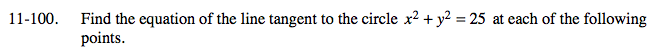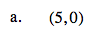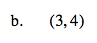### Home > A2C > Chapter Ch11 > Lesson 11.2.5 > Problem11-100

11-100.
1. Find the equation of the line tangent to the circle x2 + y2 = 25 at each of the following points. Homework Help ✎

1. (5, 0)

2. (3, 4)Find the equation of the line from the center of the circle to this point.

y = 0

Now find the equation of the line that is perpendicular to this line that passes through this point.

x = 5See part (a) for the method.

$y=-\frac{3}{4}x+\frac{25}{4}$